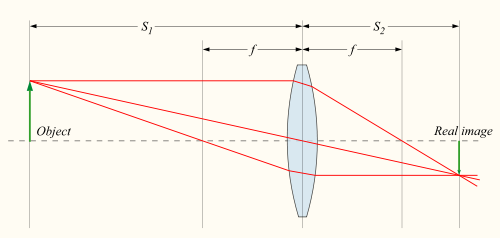How to keep a laser beam small.

My post about seeing a laser from the moon mentioned  the fact that the beam from a laser spreads as it propagates. We're used to seeing this from a flashlight - the beam from a flashlight across a room is much smaller than the beam from a flashlight across an open field. Lasers spread too, though not by as much. But spread they do, and over the quarter of a million miles to the moon, a laser will spread quite a bit. The spread of a laser (which is more accurately called its angular divergence) can be reduced though, which is useful if you want your beam diameter to be smaller at long distances. The limits of this process are worth talking about.

Let's start off with the simpler case of a magnifying glass being used to start a fire. It takes an object such as the sun, and produces an tiny but intense image of the sun on the target you're trying to ignite. For imaging generally, the distance to the object S1 and the distance to the image S2 are related to the focal length f of the lens.$\frac{1}{f} = \frac{1}{s_1} + \frac{1}{s_2}$

The magnification of the lens is given by

$m = -\frac{s_2}{s_1}$

Here the magnification is linear magnification. The sun is gigantic, the image of the sun created by the magnifying glass is a tiny brilliant pinprick of light. Therefore the magnification is actually incredibly small. We can put numbers to it by assuming that the sun is very far away compared to the focal length (ie, that 1/s1 is essentially 0 compared to 1/f.), and by writing the size of the sun as θ*s1, where θ is the angular size of the sun (about half a degree).

Do all this, and you'll find that the size of the focused image of the sun (call it d) is:

$d = -f\theta$

The minus sign just means the image is upside down. For a magnifying glass with a focal length of 15 cm, the light entering the magnifying glass is concentrated into an image of the sun just 0.13 centimeters in diameter. Shorter focal lengths mean smaller images and more concentration of the light.

The upshot of all this is that focusing involves tradeoffs. If you want a smaller and more intense spot size at the focus, you need to focus harder - the light coming out of the magnifying glass has to be directed toward the focus at a pretty sharp angle. This angle vs. size-at-focus thing is what ends up being the limiting factor for laser beam divergence too.

So take a look at this diagram from Wikipedia illustrating a laser beam being focused:The beam has a divergence angle Θ, and it has a minimum waist size w0 at the focus. Are the two related for laser beams, like they are for images? Yes indeed. It turns out that the two are related by

$\Theta \approx \frac{2\lambda}{\pi w_0}$.

Where theta is the wavelength of the light. Since the wavelength of visible light is very small, laser light can be focused down to truly tiny spot sizes resulting in ridiculous intensities.

Now for the case of sending a laser beam to the moon, we're trying to reduce the divergence angle. This means we need to increase the beam waist as far as possible.  This is usually done by sending the laser through a telescope. If the telescope has a diameter of 1 meter and you arrange for the laser beam to be expanded to that size as it leaves the telescope, the beam divergence can in principle be reduced to something on the order of a hundred-thousandths of a degree. Such a beam would have only expanded to around a few hundred meters by the time it got to the moon. While that's not a small area, it's enough for experiments such as the Apollo lunar ranging experiments.

(Personal note: sorry for vanishing from the internet over the last two weeks. I had my prelims last week, and the blog had to wait. On the plus side, I passed with no trouble and am officially All But Dissertation!)

Tags

More like this

So could you use a pair of one meter telescopes a kilometer apart to create a point of constructive interference on the moon as small as what would have been created by a kilometer size telescope?

By ppnl (not verified) on 29 Nov 2012 #permalink

I don't think so. The whole thing about interferometers having the resolution of a telescope the size of the baseline is sort of a hand-waving way of sweeping of lot of technical detail under the rug. You could make some pretty closely spaced interference fringes on the lunar surface, but you wouldn't be able to generate a bright point like you would from a kilometer-sized telescope.

No it wouldn't be as bright as what could be achieved with a kilometer sized telescope. But the central peak should be about as small as the kilometer telescope and much brighter than with a single one meter telescope. It is also scalable to many telescopes.

I think anyway. I'm not sure how to do this calculation.

By ppnl (not verified) on 30 Nov 2012 #permalink